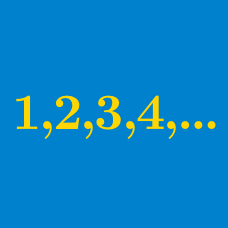Algebra

# Arithmetic Progressions: Level 4 Challenges

Find the number of strictly increasing arithmetic progressions of length three, with terms from $1$ to $1000$, that consist entirely of perfect squares.

Details and assumptions

The length of an arithmetic progression is the number of terms that it has.

There is only one arithmetic progression with 10 terms and positive difference which are all primes less than 3000.
Find the sum of the 10 terms.

150 workers are engaged to complete a job and it is known that if they all work together the job will be completed in a certain number of days. However, after the first day of work, 4 workers resign. After the second day, another 4 resign. This pattern continues until the job is finally completed, 8 days over schedule.

Find the number of days in which the work was completed.

Consider an arithmetic progression with terms $a_1, a_2, \ldots$ and the sum of the first $k$ terms is $S_k$. If

$a_{13} = \frac{1}{11} , a_{11} = \frac{1}{13},$

then find $S_{143}$.

$\sqrt{a-x}$, $\sqrt{x}$, $\sqrt{a+x}$ are first three terms of an arithmetic progression with integral terms where $a>x>0$. Find the least composite value of $a$.

×